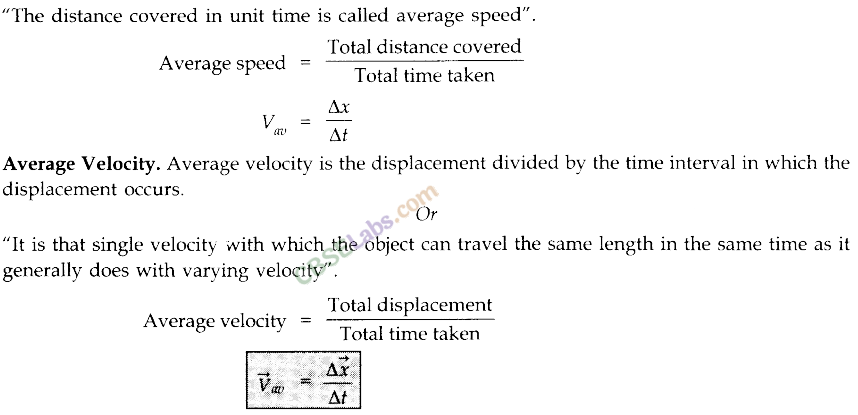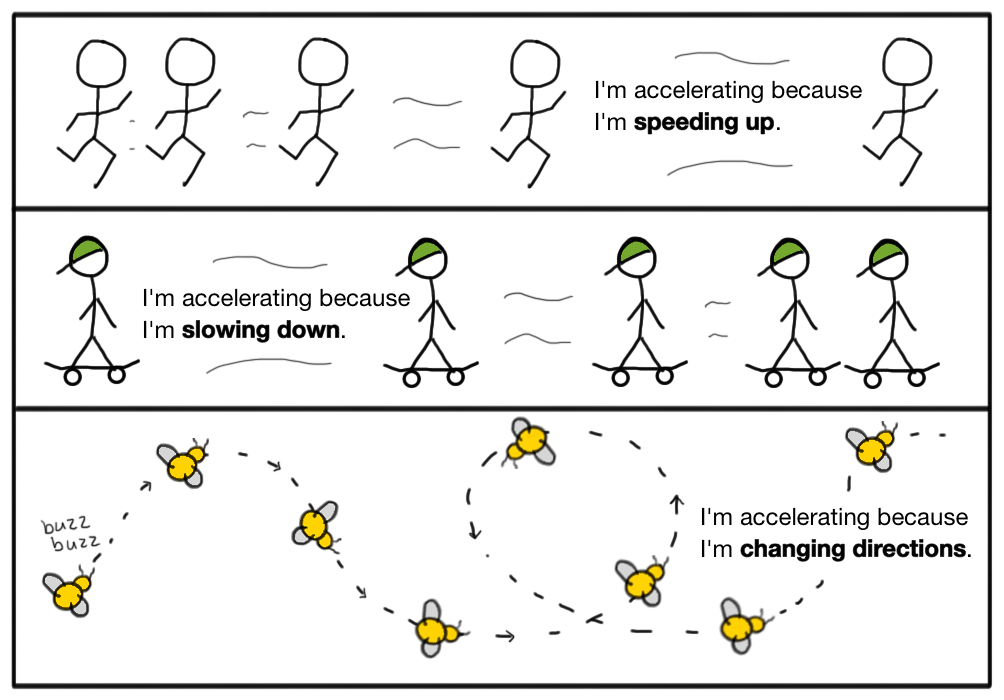# CBSE Class 11 Physics Chapter 3 Revision Notes

Chapter 3: Motion in Straight Line Revision Notes

• One of the most important topics in physics is motion.
• The universe as a whole is in motion. Although there is only a small amount of movement and it is extremely slow, movement does occur. Even if you appear to be stationary, the Earth rotates around the sun, and the sun rotates around our galaxy.Source

• If an object’s position changes over time, it is said to be in motion.
• The concept of motion is fluid, and a body that is moving relative to one reference system may be stationary relative to another.
• The study of an object’s motion is divided into two branches in physics.

(i) Kinematics: Kinematics is a branch of mathematics that studies the motion of objects without considering the cause of the motion.

(ii) Dynamics: This term refers to the relationship between object motion and the forces that cause it.

• Point Object

The objects are considered point objects if the length covered by the objects is very large in comparison to the size of the objects.

• Reference Systems

A particle’s motion is always described in terms of a reference system. A reference system is created by imagining a coordinate system attached to an arbitrary point as the origin. The reference system for a given problem is the coordinate system chosen for it. For the majority of problems, we use a coordinate system based on the earth as the reference system.

• Total Path Length

The ‘total path length’ or distance covered by a particle in motion is the total length of the actual path traversed between the particle’s initial and final positions.

## Types of Motion

• We need to specify an object’s position in order to fully describe its motion. We’ll need the position coordinates for this. Three position coordinates are required in some cases, while two or one position coordinate is required in others.
• Motion can be classified into the following categories based on these criteria:

(i) Motion in one dimension: One-dimensional motion is defined as a particle moving in a straight line or along a path. For example, a train moving in a straight line, a freely falling body under gravity, and so on.

(ii) Motion in two dimensions: Two-dimensional motion is defined as a particle moving in a plane. For example, the motion of a gun shell, carrom board coins, and so on.

(iii) Motion in three dimensions: Three-dimensional motion refers to the movement of a particle in space. For instance, the motion of a kite in the sky, the motion of an airplane, and so on.

## Displacement

• A particle’s displacement in a given time is defined as the change in its position in a specific direction during that time. A vector is drawn from its initial position to its final position to determine it.

### Distinguishing Factors Between Displacement and Distance

• Displacement moves in a specific direction. Distance has no sense of direction.
• Displacement can be positive or negative in magnitude.
• The concept of distance is always positive. It doesn’t get any smaller over time.
• Distance > |Displacement|
• Uniform Speed: If an object covers equal distances in equal intervals of time, regardless of how small these intervals of time are, it is said to move with uniform speed.
• Uniform Velocity: If an object moves with uniform velocity, it travels the same distance in the same amount of time, no matter how small the time intervals are.
• Variable Speed: If an object covers unequal distances in equal intervals of time, regardless of how small these intervals of time are, it is said to move with variable speed.
• Variable Velocity is a term that refers to the ability to change the speed If an object covers unequal displacements in equal intervals of time, regardless of how small these intervals of time are, it is said to move with variable velocity.
• Average Speed: It is the ratio of the total path length traversed to the time interval corresponding to it.Source

• The magnitude of the average velocity over a given time interval is greater than or equal to the average speed of an object.
• Instantaneous Speed is a term used to describe the speed with which something happens. Instantaneous speed is the speed of an object at a specific point in time.
• Instantaneous Velocity: A particle’s instantaneous velocity is its speed at any given point in time or along its path.
• Acceleration: Acceleration is the rate at which velocity changes.Source

• Uniform Acceleration: Uniform acceleration occurs when an object experiences equal changes in velocity at equal time intervals.
• Average Acceleration: It is calculated by dividing the change in velocity by the time interval during which the change occurs.
• Instantaneous Acceleration is a term used to describe a sudden increase in speed. It is defined as the average acceleration’s limit as the time interval t approaches zero.
]]>